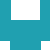#### [KS4][(i)GCSE][Higher][Revision][Workbook] Year 10Year 11KS 4

2020-5-1 314

Number Skills
1. Rational and irrational numbers
2. Checking
Calculator Skills
3. Using a calculator: Brackets and memory
4. Using a calculator: Powers, roots, memory
5. Standard form – 1
6. Standard form – 2
Fractions, Decimals and Percentages
7. Percentages and fractions
8. Calculating growth and decay rates
Number Patterns
9. Patterns you must recognise
10. Product of primes, highest common factor, lowest common multiple, reciprocals
11. Trial and improvement
Equations
12. Equations
13. Rewriting formulae
14. Iteration
Variation
15. Direct and inverse variation
Algebraic Skills
16. Using algebraic formulae
17. Rules for indices (powers)
18. Expansion of brackets
19. Factorisation – 1
20. Factorisation – 2
21. Factorisation – 3
22. Factorisation – 4
23. Factorisation – 5
25. Simultaneous equations: Solving using algebra – 1
26. Simultaneous equations: Solving using algebra – 2
27. Simplifying algebraic fractions – 1
28. Simplifying algebraic fractions – 2
Graphs
29. Drawing lines
30. Simultaneous equations: Solving by drawing a graph – 1
31. Simultaneous equations: Solving by drawing a graph – 2
32. Solving equations using graphical methods
33. The straight line equation – 1
34. The straight line equation – 2
35. Using tangents to find gradients
36. Expressing general rules in symbolic form – 1
37. Expressing general rules in symbolic form – 2
38. Inequalities – 1
39. Inequalities – 2
40. Drawing graphs – 1
41. Drawing graphs – 2
42. Sketching graphs – 1
43. Sketching graphs – 2
44. Speed, time and distance graphs
45. Area under a curve
Angles
46. Intersecting and parallel lines
47. Bearings – 1
48. Bearings – 2
49. Angles
Similarity
50. Similarity – 1
51. Similarity – 2
Congruence
52. Congruent triangles – 1
53. Congruent triangles – 2
Transformations
54. Combined and inverse transformations
55. Enlargement by a fractional scale factor
56. Enlargement by a negative scale factor
57. Translations
Measurement
58. Compound measures
59. Time
60. Upper and lower bounds – 1
61. Upper and lower bounds – 2
Circles
62. Length, area and volume of shapes with curves
63. Angle and tangent properties of circles – 1
64. Angle and tangent properties of circles – 2
65. Angle and tangent properties of circles – 3
Perimeter, Area and Volume
66. Calculating length, area and volume – 1
67. Calculating length, area and volume – 2
68. Calculating length, area and volume – 3
69. Formulae for length, area and volume
70. Ratio for length, area and volume
Pythagoras' Theorem and Trigonometry
71. Pythagoras’ theorem
72. Trigonometry: Finding an angle – 1
73. Trigonometry: Finding an angle – 2
74. Trigonometry: Finding a side – 1
75. Trigonometry: Finding a side – 2
76. Trigonometry: Solving problems – 1
77. Trigonometry: Solving problems – 2
78. Trigonometry and Pythagoras' theorem in 3-D shapes
79. Sine, cosine and tangent of any angle – 1
80. Sine, cosine and tangent of any angle – 2
81. Sine, cosine and tangent of any angle – 3
82. Sine rule, cosine rule, area of a triangle – 1
83. Sine rule, cosine rule, area of a triangle – 2
Vectors
84. Vectors – 1
85. Vectors – 2
86. Vectors – 3
87. Vectors – 4
Locus
88. Locus
Questionnaires
89. Designing questionnaires
90. Sampling
91. Hypotheses
Tables and Graphs
92. Comparing data
93. Histograms
94. Grouped data
Cumulative Frequency
95. Cumulative frequency – 1
96. Cumulative frequency – 2
97. Using cumulative frequency diagrams to compare data – 1
98. Using cumulative frequency diagrams to compare data – 2
Scatter Diagrams
99. Line of best fit
Probability
100. Estimate of probability by experiment
101. Tree diagrams – 1
102. Tree diagrams – 2
103. Conditional and independent probability
104. Probability: And/or
105. Probability: At least – 1
106. Probability: At least – 2
107. Probability: Examination – type questions